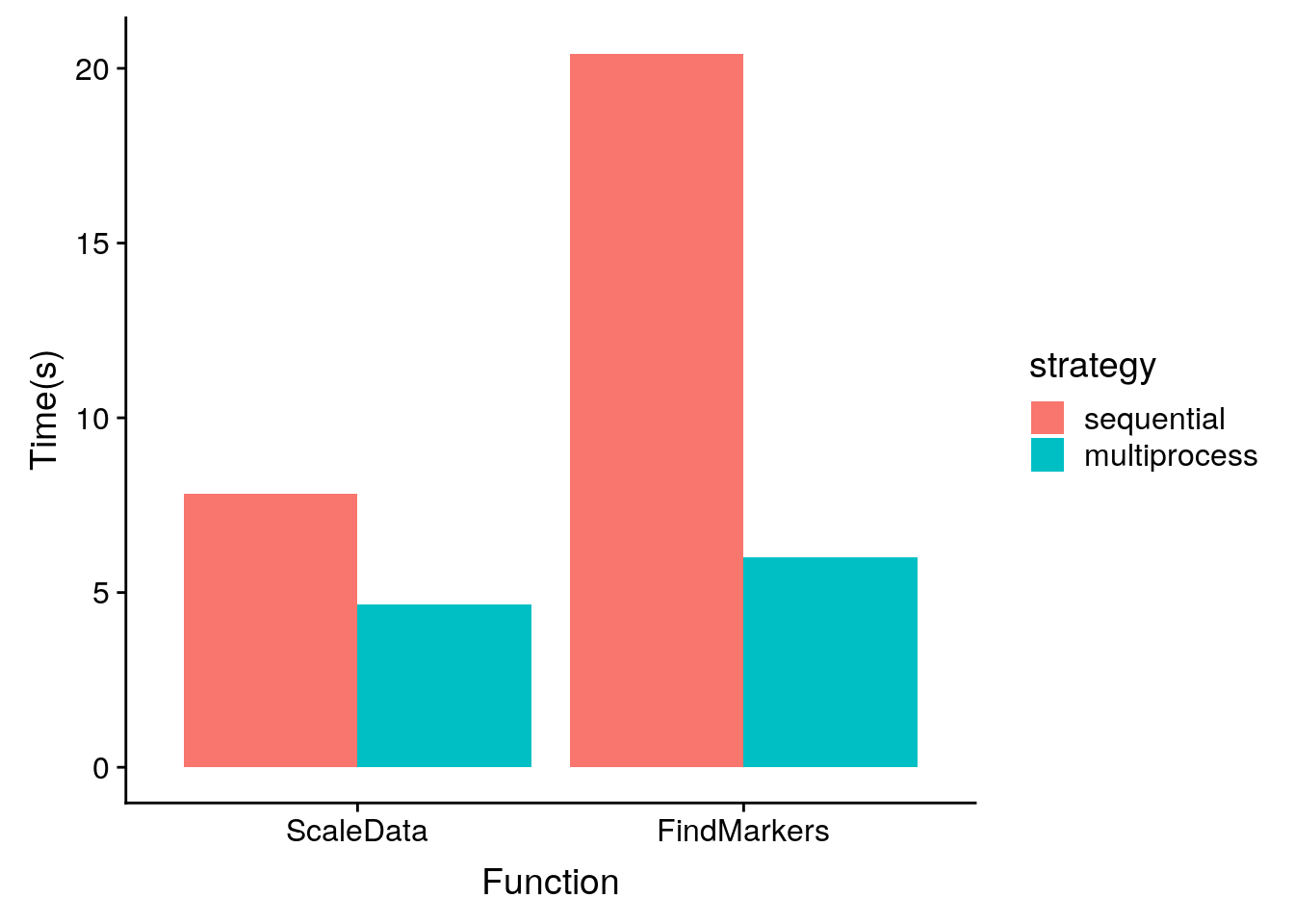In Seurat, we have chosen to use the future framework for parallelization. In this vignette, we will demonstrate how you can take advantage of the future implementation of certain Seurat functions from a user’s perspective. If you are interested in learning more about the future framework beyond what is described here, please see the package vignettes here for a comprehensive and detailed description.

### How to use parallelization in Seurat

To access the parallel version of functions in Seurat, you need to load the future package and set the plan. The plan will specify how the function is executed. The default behavior is to evaluate in a non-parallelized fashion (sequentially). To achieve parallel (asynchronous) behavior, we typically recommend the “multiprocess” strategy. By default, this uses all available cores but you can set the workers parameter to limit the number of concurrently active futures.

library(future)
# check the current active plan
plan()
## sequential:
## - args: function (expr, envir = parent.frame(), substitute = TRUE, lazy = FALSE, seed = NULL, globals = TRUE, local = TRUE, earlySignal = FALSE, label = NULL, ...)
## - tweaked: FALSE
## - call: plan("default", .init = FALSE)
# change the current plan to access parallelization
plan("multiprocess", workers = 4)
plan()
## multiprocess:
## - args: function (expr, envir = parent.frame(), substitute = TRUE, lazy = FALSE, seed = NULL, globals = TRUE, workers = 4, gc = FALSE, earlySignal = FALSE, label = NULL, ...)
## - tweaked: TRUE
## - call: plan("multiprocess", workers = 4)

### ‘Futurized’ functions in Seurat

The following functions have been written to take advantage of the future framework and will be parallelized if the current plan is set appropriately. Importantly, the way you call the function shouldn’t change.

• NormalizeData
• ScaleData
• JackStraw
• FindMarkers
• FindIntegrationAnchors
• FindClusters - if clustering over multiple resolutions

For example, to run the parallel version of FindMarkers, you simply need to set the plan and call the function as usual.

library(Seurat)

# Enable parallelization
plan("multiprocess", workers = 4)
markers <- FindMarkers(pbmc, ident.1 = "NK", verbose = FALSE)

### Comparison of sequential vs. parallel

Here we’ll perform a brief comparison the runtimes for the same function calls with and without parallelization. Note that while we expect that using a parallelized strategy will decrease the runtimes of the functions listed above, the magnitude of that decrease will depend on many factors (e.g. the size of the dataset, the number of workers, specs of the system, the future strategy, etc). The following benchmarks were performed on a desktop computer running Ubuntu 16.04.5 LTS with an Intel(R) Core(TM) i7-6800K CPU @ 3.40GHz and 96 GB of RAM.

Click to see bencharking code

library(cowplot)
timing.comparisons <- data.frame(fxn = character(), time = numeric(), strategy = character())
plan("sequential")
start <- Sys.time()
pbmc <- ScaleData(pbmc, vars.to.regress = "percent.mt", verbose = FALSE)
end <- Sys.time()
timing.comparisons <- rbind(timing.comparisons, data.frame(fxn = "ScaleData", time = as.numeric(end -
start, units = "secs"), strategy = "sequential"))

start <- Sys.time()
markers <- FindMarkers(pbmc, ident.1 = "NK", verbose = FALSE)
end <- Sys.time()
timing.comparisons <- rbind(timing.comparisons, data.frame(fxn = "FindMarkers", time = as.numeric(end -
start, units = "secs"), strategy = "sequential"))

plan("multiprocess", workers = 4)
start <- Sys.time()
pbmc <- ScaleData(pbmc, vars.to.regress = "percent.mt", verbose = FALSE)
end <- Sys.time()
timing.comparisons <- rbind(timing.comparisons, data.frame(fxn = "ScaleData", time = as.numeric(end -
start, units = "secs"), strategy = "multiprocess"))

start <- Sys.time()
markers <- FindMarkers(pbmc, ident.1 = "NK", verbose = FALSE)
end <- Sys.time()
timing.comparisons <- rbind(timing.comparisons, data.frame(fxn = "FindMarkers", time = as.numeric(end -
start, units = "secs"), strategy = "multiprocess"))
ggplot(timing.comparisons, aes(fxn, time)) + geom_bar(aes(fill = strategy), stat = "identity", position = "dodge") +
ylab("Time(s)") + xlab("Function")Unfortantely, the when running these functions in any of the parallel plan modes you will lose the progress bar. This is due to some technical limitations in the future framework and R generally. If you want to monitor function progress, you’ll need to forgo parallelization and use plan("sequential").
Error in getGlobalsAndPackages(expr, envir = envir, globals = TRUE) :
The total size of the X globals that need to be exported for the future expression ('FUN()') is X GiB. This exceeds the maximum allowed size of 500.00 MiB (option 'future.globals.maxSize'). The X largest globals are ... 
For certain functions, each worker needs access to certain global variables. If these are larger than the default limit, you will see this error. To get around this, you can set options(future.globals.maxSize = X), where X is the maximum allowed size in bytes. So to set it to 1GB, you would run options(future.globals.maxSize = 1000 * 1024^2). Note that this will increase your RAM usage so set this number mindfully.